# ISEE Upper Level Math : Tetrahedrons

## Example Questions

← Previous 1 2 Next →

### Example Question #1 : How To Find The Surface Area Of A Tetrahedron

In three-dimensional space, the four vertices of a tetrahedron - a solid with four faces - have Cartesian coordinates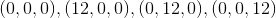.

What is the surface area of this tetrahedron?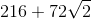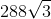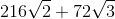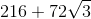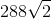Explanation:

The tetrahedron looks like this: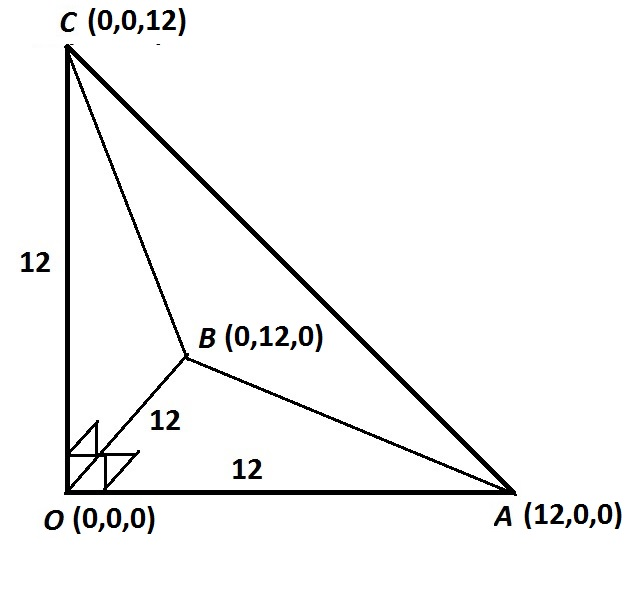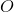is the origin and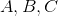are the other three points, which are each twelve units away from the origin on one of the three (mutually perpendicular) axes.

Three of the surfaces are right triangles with two legs of length 12, so the area of each is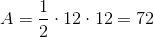.

The fourth surface,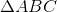, has three edges each of which is the hypotenuse of an isosceles right triangle with legs 12, so each has length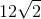by the 45-45-90 Theorem. That makes this triangle equilateral, so its area is'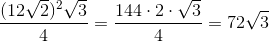The surface area is therefore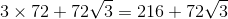.

### Example Question #2 : How To Find The Surface Area Of A Tetrahedron

In three-dimensional space, the four vertices of a tetrahedron - a solid with four faces - have Cartesian coordinates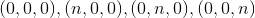.

In terms of, give the surface area of this tetrahedron.Explanation:

The tetrahedron looks like this: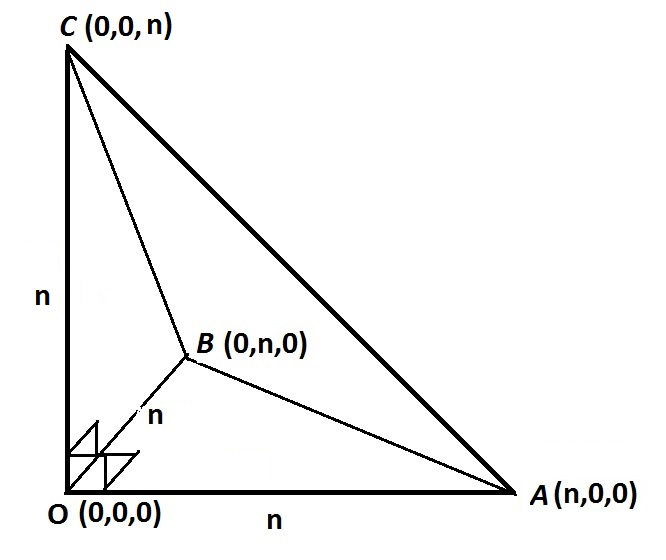is the origin andare the other three points, which areunits away from the origin, each along one of the three (perpendicular) axes.

Three of the surfaces are right triangles with two legs of length 12, so the area of each is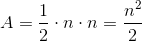.

The fourth surface,, has three edges each of which is the hypotenuse of an isosceles right triangle with legs, so each has length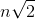by the 45-45-90 Theorem. That makes this triangle equilateral, so its area is'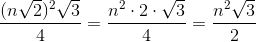The surface area is therefore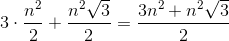.

← Previous 1 2 Next →

### All ISEE Upper Level Math Resources